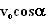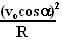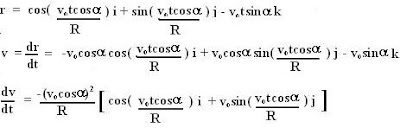## Friday, November 23, 2007

### Irodov Problem 1.94Viewed from the point of a stationary observer, the mass turns along the cylinder because of the Normal reaction N offered by the surface of the cylinder. This normal reaction is the same force that pushes the cylinder outwards as well. We shall solve this problem in two ways i) using the knowledge of centripetal acceleration and ii) mathematically. This will help understand the origin of centripetal acceleration.

Method 1 : Using Centripetal Acceleration The body experiences centripetal acceleration radially inwards as it rotates. The component of the tangential velocity in the direction of the normal reaction isand thus the centripetal acceleration is. There is no other acceleration along this direction. Thus from newton's laws we have,Method 2 : Mathematically
If there were no gravity, the position the particle vectorially is given by,This is basically the centripetal acceleration directed radially inwards. The normal reaction N also is directed radially inwards as sown in the Figure. Even if there were gravity acting on the particle, there would be no component of gravity along the direction of centripetal acceleration which is directed radially inwards along the direction of the Normal reaction N, and hence would not change the answer. Now the rest of the solution is simply as in Method 1.